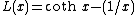# Langevin function

## Langevin function

A mathematical function which is important in the theory of paramagnetism and in the theory of the dielectric properties of insulators. The analytical expression for the Langevin functionis shown in the equation below. If . The paramagnetic susceptibility of a classical (non-quantum-mechanical) collection of magnetic dipoles is given by the Langevin function, as is the polarizability of molecules having a permanent electric dipole moment. See Paramagnetism

McGraw-Hill Concise Encyclopedia of Physics. © 2002 by The McGraw-Hill Companies, Inc.

## Langevin function

[länzh·van ‚fəŋk·shən]
(electromagnetism)
A mathematical function, L (x), which occurs in the expressions for the paramagnetic susceptibility of a classical (non-quantum-mechanical) collection of magnetic dipoles, and for the polarizability of molecules having a permanent electric dipole moment; given by L (x) = coth x- 1/ x.
McGraw-Hill Dictionary of Scientific & Technical Terms, 6E, Copyright © 2003 by The McGraw-Hill Companies, Inc.
References in periodicals archive ?
with L([xi]) being Langevin function and [H.sub.e] having the meaning of an effective magnetic field that acts on each monodomenic particle.
The energy of dipolar interactions is considered lower than the thermal energy and the equilibrium magnetization is written as a superposition of Langevin functions. Also, the linear dependence of the initial magnetic susceptibility on magnetic nanoparticles concentration, in low fields ,
The Langevin function, L(x) = coth(v) - 1/x, gives the magnitude of the magnetization in thermal equilibrium.
instead of the Langevin function appearing in (10).
Figure 1 shows that this equilibrium magnetization is determined by the Langevin function (L) and Rosensweig's chord susceptibility (R).
As we take into account the curvature of the Langevin function, we can also discuss the implications of the chord susceptibility.
To avoid the difficult integral, for [zeta] [much less than] 1 one can take the susceptibility instead of the Langevin function, that is, the first term in the Taylor series of L, that is, the Curie susceptibility, instead of the function L.
Keeping only the k = 0 terms in the second sum in (33) amounts to eliminating the higher harmonics in the time dependence of the magnetization, which are generated due to the substantial nonlinearity of the Langevin function beyond x [approximately equal to] 0.5.
The SEF, [omega], for the eight chain model is a series expansion of the inverse Langevin function, [L.sup.-1], and can be written in summation form as
Horgan and Saccomandi  demonstrated that the Gent model produced a close approximation to the inverse Langevin function, [L.sup.-1].
Site: Follow: Share:
Open / Close